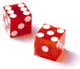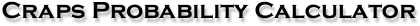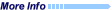Type of Bet:
 Pass Line Don't Pass Place 6/8 Place 5/9 Place 4/10Field* Hard 6/8 Hard 4/10 Any Craps Any Seven
What is the probability of consecutive ?
What is the probability that exactly decisions
will
out of total decisons?
What is the probability that or
decisions will
out of total decisons?NotesDisclaimer: these are only probabilities, they do not guarantee what will happen at an actual table!Usage: you just need to select the type of bet (Pass Line is the default), and then fill in the blanks for one (or several) of the following three questions.  Specify the number of wins/losses and a total number of decisions – for example, you could request the probability of 22 losses or more out of a total of 26 decisions on the Don't Pass, which equals .0003443, or about 1 chance in 2904.  This is the probability, out of a total of 26 decisions, of either 22, 23, 24, 25, or 26 losses (the order of the wins and losses are irrelevant).  The probability of 22 or more losses is equivalent to the probability of 5 or fewer wins.Also remember that if you calculate a certain result over, say, 10 total decisions, and you get a probability of 1 in 1000, the probability refers to 1 in 1000 sets of 10 decisions (10,000 decisions).If you make a calculation using sufficiently large numbers, you will get scientific notation as your result (e.g., 5.084e-8 = .00000005084); simply move the period 8 digits to the left in the above example (or right, if e is positive).For Line bets, the calculation does not take odds into account – if you take odds, the probability applies to the flat bet only!*Refers to Field bets with a double two and twelve, not a triple two or twelve (which can be found in some casinos and have a lower house edge).